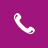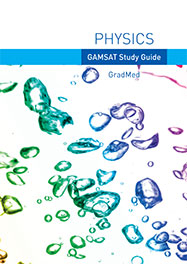020 7584 5818# Physics

## Syllabus

• Basics - S.I. system of units, scalars and vectors.
• Rectilinear Motion - velocity and acceleration, graphs of motion, equations of motion.
• Forces - resultant forces, weight and mass, resolving forces, centres of gravity, upthrust and flotation, moments, Newton’s laws.
• Dynamics - projectiles, momentum, circular motion.
• Energy - forms of energy, energy transfers, conservation of energy, KE and PE and associated formulae, power.
• Gases - The gas laws, ideal gas, absolute temperature scale, meaning of temperature.
• Thermal energy - transfer (conduction, convection and radiation), heat capacity, latent heat, thermodynamics.
• Electric circuits - current and potential difference, combinations of resistances, resistivity, EMF, internal resistance, capacitors and capacitor equations.
• Electric fields - Coulomb’s law, comparison with gravitational fields.
• Magnetic fields - electromagnetism (uses and formula), mass spectrometry.
• Waves - mechanical waves, wave phenomena
• Optics - refractive index, lenses and lens formulae, focal lengths, real and virtual images, the eye (application of the above topic to the eye and defects of vision).
• Simple Harmonic Motion - formulation, resonance, damped oscillations.
• Sound - intensity, logarithm dB scale.
• Radioactivity - nuclear decay (alpha, beta, gamma), exponential decay, half-life
• Nuclear reactions - mass-energy equivalence, fission and fusion.
• Medical physics - x-rays, ultrasound, optics in medicine, radio-isotopes, Magnetic Resonance Imaging.

Where possible, topics are covered so that the following aspects are stressed

• Analysis and interpretation of graphs
• Mathematical patterns (direct and inverse proportionality, exponential changes, log scales etc).
• Quick approaches to solutions.
• Conversions of simple (eg: µA to mA) and more complex units (eg: g cm-3 to kg m-3).

It is important to note that these cross-curricular techniques will also serve to reinforce your work in the other sciences.